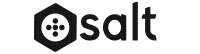#Imagine the simplicity of following the most basic of mathematical equations, like 2 + 2. From young to old, we agree on the answer.

We need a similar formula that can be simplified for the development of leaders. What can we do to simplify the equation?

Here are a few suggestions.

1) Desire: Without this step, leaders cannot be developed.
2) Ability: A system of measurement must be in place.